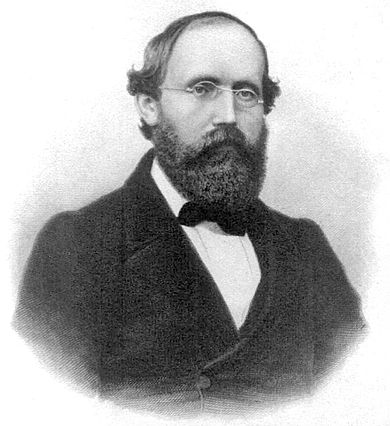Georg Friedrich Bernhard Riemann 1826-1866

# Introduction

Bernhard Riemann was a German mathematician who made significant contributions to analysis, number theory, and differential geometry. He is best known for his work on the theory of Riemann surfaces, which helped to establish the modern field of complex analysis, and for his contributions to the study of multi-dimensional spaces.

# Early life

• Born: September 17, 1826 in Breselenz, Germany
• Showed an early aptitude for mathematics and was accepted into the University of Göttingen at the age of 19.

• Studied at the University of Göttingen under leading mathematicians including Carl Friedrich Gauss and Peter Gustav Lejeune Dirichlet.
• Became a professor at the University of Göttingen and later at the University of Berlin.

# Major contributions to mathematics

Bernhard Riemann made several significant contributions to mathematics throughout his career. Some of his most important theorems and concepts include:

• The Riemann integral, which allows for the definition of integrals of functions that are not necessarily continuous. The Riemann integral is defined as the limit of a sequence of Riemann sums, which are constructed by dividing the interval of integration into subintervals and approximating the function by a piecewise constant function. This construction allows for the integration of functions that may not have a well-defined value at every point in the interval, and is a key tool in the study of real analysis.

• The Riemann-Roch theorem, which relates the number of independent solutions to a system of algebraic equations to the topological properties of the solutions. The theorem is used in the study of algebraic curves and has important applications in geometry and topology.

Let $C$ be a compact Riemann surface of genus $g$, and let $D$ be a divisor on $C$. Then the Riemann-Roch theorem states that:

$\text{dim}(L(D)) - \text{dim}(L(-D)) = \text{deg}(D) + 1 - g$

where $L(D)$ denotes the space of meromorphic functions on $C$ with poles of at most order 1 at the points of $D$, and $\text{dim}(L(D))$ denotes the dimension of this space.

In this statement, the term $\text{deg}(D)$ denotes the degree of the divisor $D$, which is defined as the sum of the orders of the points in $D$. The term $g$ is the genus of the Riemann surface $C$, which is a topological invariant that measures the number of “holes” in the surface.

• The concept of a Riemannian manifold, which is a type of multi-dimensional space that is equipped with a metric tensor that allows for the definition of distance and angles between points. Riemannian manifolds are an important tool in the study of geometry and are used to model physical phenomena such as the curvature of space-time in general relativity.

A Riemannian manifold is a smooth manifold $M$ equipped with a smooth, symmetric, positive definite inner product $g_{ab}$ on each tangent space $T_pM$, where $p \in M$.

This inner product allows us to define the length of a tangent vector $v \in T_pM$ as:

$\left\|v\right\| = \sqrt{g_{ab}v^av^b}$

and the angle between two tangent vectors $u, v \in T_pM$ as:

$\cos \theta = \frac{g_{ab}u^av^b}{\left\|u\right\|\left\|v\right\|}$

where $u^a$ and $v^a$ are the components of $u$ and $v$ with respect to a local coordinate system on $M$.

The metric tensor $g_{ab}$ encodes information about the geometry of the manifold and can be used to define various geometric quantities, such as distances and angles between points, curvature, and volume. Riemannian manifolds are used in various areas of mathematics and physics to model the geometry of curved spaces and to study the behavior of physical phenomena in these spaces.

• The Riemann curvature tensor, which is a mathematical object that describes the curvature of a Riemannian manifold. The curvature tensor is used to measure the deviation of the manifold from flatness and is an important tool in the study of the geometry of curved spaces.

Let $(M, g_{ab})$ be a Riemannian manifold. The Riemann curvature tensor is a multilinear map

$R : T_pM \times T_pM \times T_pM \rightarrow T_pM$

defined at each point $p \in M$ by:

$R(u, v)w = \nabla_u \nabla_v w - \nabla_v \nabla_u w - \nabla_{[u, v]}w$

where $u, v, w \in T_pM$ are tangent vectors, $\nabla$ is the Levi-Civita connection associated with the metric $g_{ab}$, and $[u, v]$ denotes the Lie bracket of $u$ and $v$.

The components of the Riemann curvature tensor in a local coordinate system $(x^1, \dots, x^n)$ on $M$ are given by:

$R_{ijkl} = g_{ia}R(e_j, e_k)e_l^a$

where $e_1, \dots, e_n$ is a local frame on $M$, and $e_i^a$ are the components of $e_i$ with respect to the frame.

The Riemann curvature tensor encodes information about the curvature of the Riemannian manifold and is used to study the geometry of curved spaces. It is a fundamental object in differential geometry and has applications in various areas of mathematics and physics.

Bernhard Riemann’s work on these and other concepts and theorems has had a lasting impact on mathematics and his ideas continue to be studied and developed by researchers today.

# Later life and legacy

• Died on July 20, 1866 at the age of 39 due to complications from tuberculosis.
• Despite his relatively short career, Riemann’s work has had a lasting impact on mathematics and his contributions continue to be studied and built upon by mathematicians today.
• Recognized as one of the greatest mathematicians in history and his name is associated with many important concepts and theorems in mathematics.

# Conclusion

Bernhard Riemann was a pioneering mathematician whose contributions to the fields of analysis, number theory, and differential geometry have had a lasting impact on the field. His work on the theory of Riemann surfaces and the concept of multi-dimensional spaces continue to be fundamental to modern mathematics and his ideas continue to be studied and developed by researchers today.